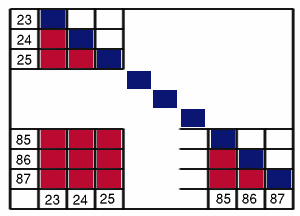#### You may also like### Double Digit### Upsetting Pitagoras

Find the smallest integer solution to the equation 1/x^2 + 1/y^2 = 1/z^2### Rudolff's Problem

A group of 20 people pay a total of £20 to see an exhibition. The admission price is £3 for men, £2 for women and 50p for children. How many men, women and children are there in the group?

# Times Right

##### Age 11 to 16Challenge Level

Students from Jack Hunt School Peterborough called this "the blank boxes puzzle" and sent lots of good solutions including some beautifully word processed work:

64 x 58 = 3712 (Kenny)

37 x 58 = 2146 (Daniel)

24 x 57 = 1368 (Adnan)

34 x 52 = 1768 (Freddie)



James, Sheherazade, Nicola and Elizabeth from Jack Hunt School, Peterboroughand Larissa, Hollie, Emma, Emma, Laura, and Lyndsay from The Mount School, York all gave excellent explanations of how to use logical thinking and be systematic in searching for solutions.

James provided a diagram highlighting the pairs of numbers he could eliminate according to the following rules. James reduced the number of cases he had to check by multiplication and found the four solutions given above. In his diagram all the numbers from 23 to 87 are included and the cells are coloured in as the number pairs are eliminated.1. Start checking numbers at 23 because numbers starting with 1 (such as 19 x 23) do not give a four figure answer or give a four figure number starting with 1 which is a repeat.
2. Take only numbers below the blue line, on the right hand side of the blue line pairs of numbers are repeated (e.g. 34 x 26 is the same as 26 x 34).
3. Numbers on the blue diagonal line are not included, they consist of pairs of the same number (e.g.23 x 23).
4. Highlight numbers which have a digit used twice in the product (e.g. 24 x 32 or 23 x 24 or 37 x 74). Don't multiply any numbers in the same decade (Sheherazade's rule).
5. Numbers ending in a 1 are eliminated because they always duplicate the last digit of the number they are multiplying (e.g. 71 x 43 = 3053, 71 x 62 = 4402)
6. Numbers ending in a 5 are eliminated because they always produce an answer that either duplicates the digit 5 or produces a zero which is not wanted.
7. I highlighted numbers which I eliminated by multiplying the units digits in my head and seeing a duplication in the units (e.g 87 x 247 x 4 = 28 repeating the 8).

Beth from The Mount School, York found two solutions for the numbers 2 to 9:

53 x 92=4876

62 x 87=5394



Are there any others? Are there any solutions for digits 0 to 7?